maths > wholenumbers

what you'll learn...

overview

This page introduces addition of whole numbers as combining two quantities and counting or measuring the combined quantity.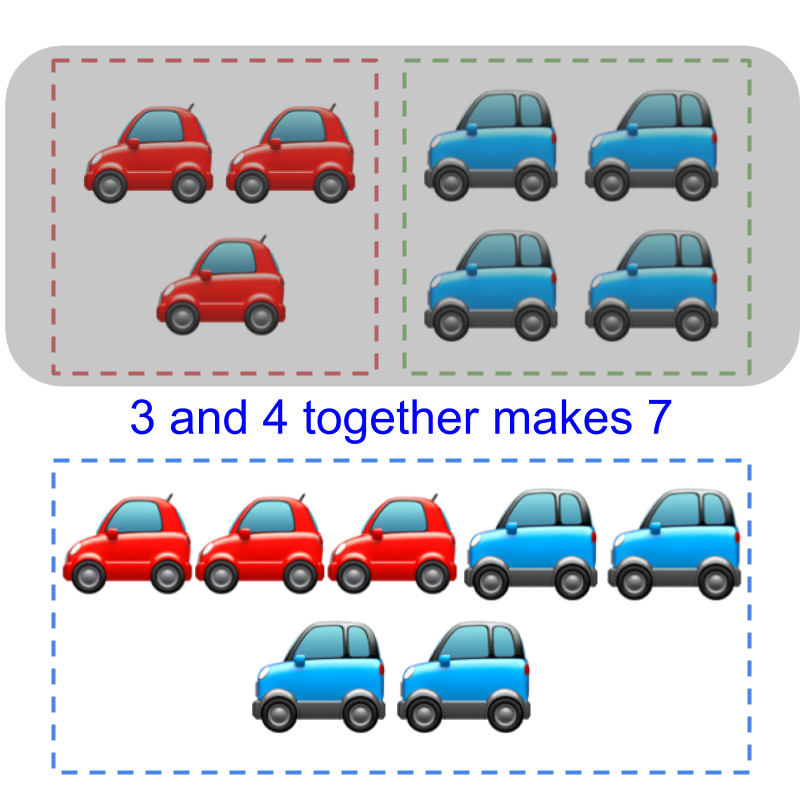Counts of 3$3$ and 4$4$ are combined together and the counting is done from 1$1$ to 7$7$. The resulting count is 7$7$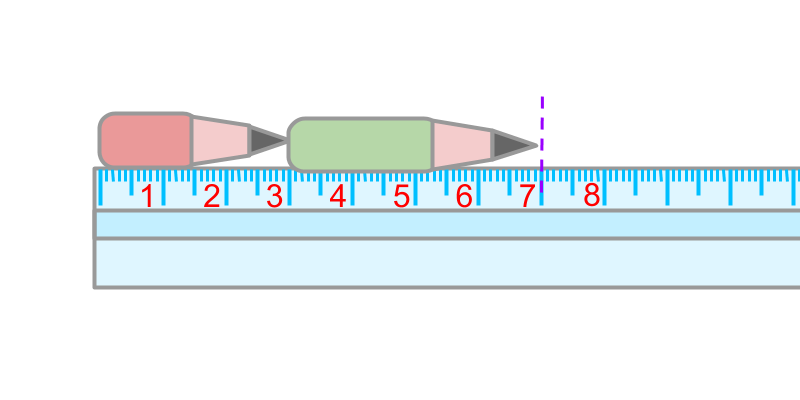Measures of 3$3$ and $4$ are combined together. The measure of combined quantity is $7$

The same is abstracted for addition in number-line.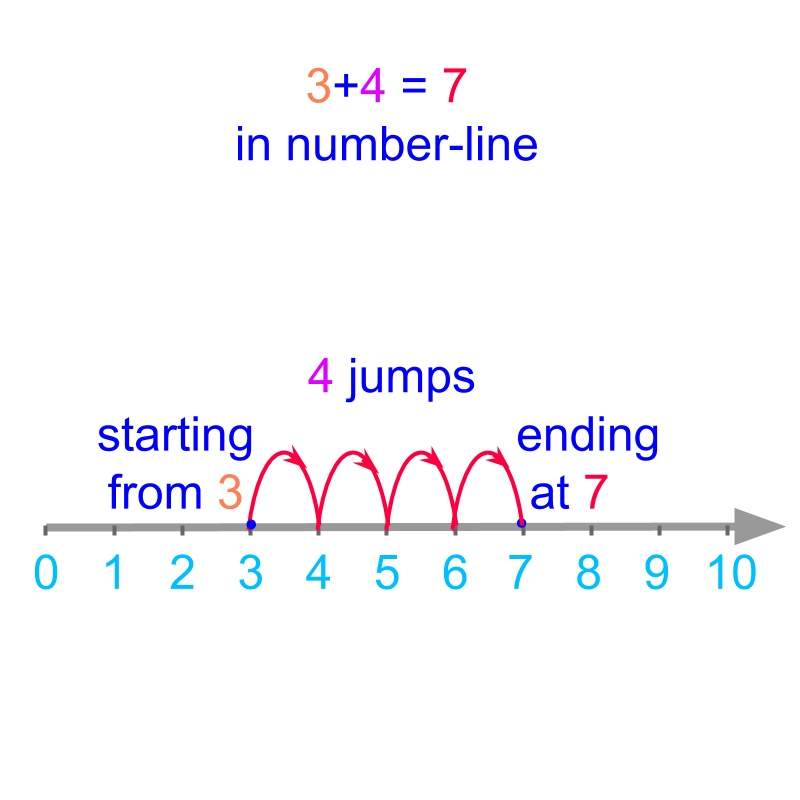The definition of addition in first principles forms the basis to understanding the simplified procedure for addition of large numbers.

combine them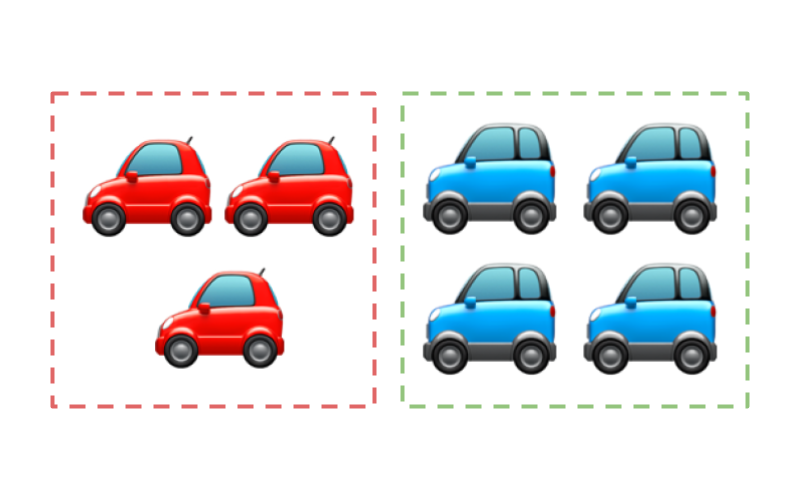There are $3$ red cars and $4$ blue cars in the figure. These cars are combined together and the total number of cars are counted.Considering the figure with $3$ red cars and $4$ blue cars. $3$ and $4$ are combined together and the counting is done from $1$ to $7$. The resulting count is $7$.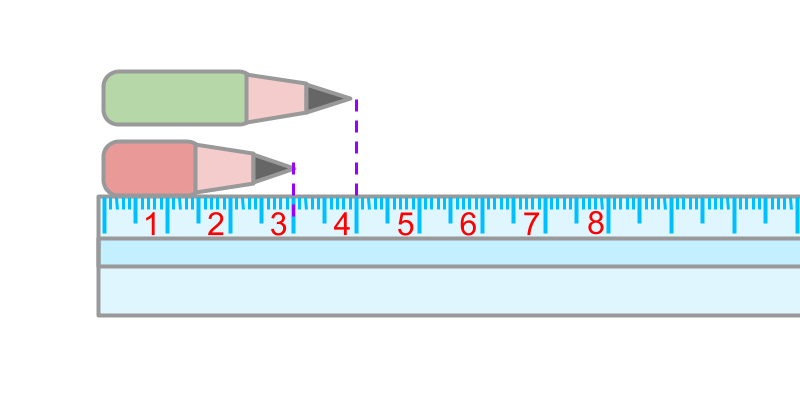A red pencil is $3$ centimeters in length. A green pencil is $4$ centimeters in length.The two pencils are placed side-by-side. The length measurements is taken from
$0$ and the measure at the end is $7$.

The count or measure of quantities can be combined or added.

The addition results in count or measure of the combined quantity.Addition $3 + 4$ is given in number-line. The count is started from $3$. $4$ jumps are made to land in $7$. The result of the addition is $7$.

The English word addition means: combining two quantities and measuring or counting the combined quantity.

Addition - First Principles : Two numbers are considered, each of which represents a count or measurement. The quantities represented by the numbers are combined to form a result representing a combined count or measurement. The combined count or measurement is the result of addition.

eg: $20$ and $13$ are combined together $20 + 13 = 33$.

$20$ is an addend

$13$ is also an addend

$33$ is the sum

sum is the result of the addition.

What is the sum of $4$ and $2$?
The answer is '$6$'.

summary

Addition - First Principles : Two numbers are considered, each of which represents a count or measurement. The quantities represented by the numbers are combined to form a result representing a combined count or measurement. The combined count or measurement is the result of addition.

eg: $20$ and $13$ are combined together $20 + 13 = 33$.

$20$ is an addend

$13$ is also an addend

$33$ is the sum

Outline